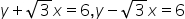Maths-
General
Easy

###Hint:

In this question, we have to find the equation of the circumcircle of the triangle formed by the linesand y=0. Let the equations be the equation of lines of triangle ABC. Considering AB and BC to find the point B as it is the common point. Similarly, finding point C using AC and BC and finding A using AB and AC. Now we have the co-ordinates of points A, B and C. Further, assuming P (h, k) to be the center of the circle, so from AP = CP and BP = CP we will get two equations using which we can find the co-ordinates of P. Now using a point from A, B or C and P we can find the radius. Further we have the co-ordinates of center and the radius, so we can find the equation of the circle.

## Book A Free Demo+91